# Irregular prime number

(diff) ← Older revision | Latest revision (diff) | Newer revision → (diff)

An odd prime numberfor which the number of classes of ideals in the cyclotomic field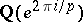is divisible by. All other odd prime numbers are called regular.

Kummer's test allows one to solve for each given prime number the problem of whether it is regular or not: For an odd prime number to be regular it is necessary and sufficient that none of the numerators of the first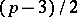Bernoulli numbers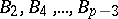is divisible by(cf. ).

The problem of the distribution of regular and irregular prime numbers arose in this connection. Tables of the Bernoulli numbers and Kummer's test indicated that among the first hundred there are only three irregular prime numbers, 37, 59, 67 (the numerators of,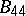and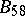are multiples of 37, 59 and 67, respectively). E. Kummer conjectured that there are on the average twice as many regular prime numbers as irregular ones. Later C.L. Siegel  conjectured that the ratio of irregular prime numbers to regular prime numbers contained in an interval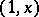tends to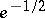as(hereis the base of natural logarithms). Up till now (1989) it is only known that the number of irregular prime numbers is infinite. There are 439 regular and 285 irregular prime numbers among the odd numbers smaller than 5500, cf. .

For any regularthe Fermat equationdoes not have non-zero solutions in the rational numbers .

Letbe an irregular prime number, let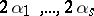be the indices of the Bernoulli numbers amongwhose numerators are divisible byand letandbe natural numbers such that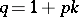is a prime number smaller than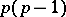and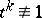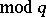. Let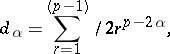If for each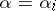,,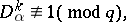then for the irregular prime numberFermat's theorem holds, i.e. the Fermat equation is unsolvable in the non-zero rational numbers. This is called Vandiver's test. The truth of Fermat's theorem for all exponents smaller than 5500 has been proved by using Vandiver's test (cf. ).

How to Cite This Entry:
Irregular prime number. Encyclopedia of Mathematics. URL: http://encyclopediaofmath.org/index.php?title=Irregular_prime_number&oldid=18479
This article was adapted from an original article by V.A. Dem'yanenko (originator), which appeared in Encyclopedia of Mathematics - ISBN 1402006098. See original article